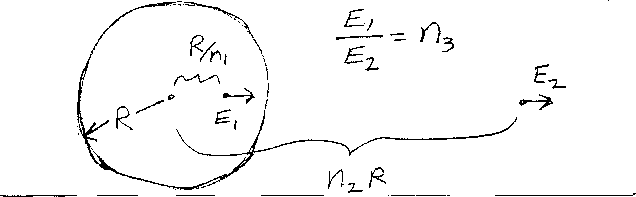Problem A11: In the figure above, there is a uniformly charged non-conducting sphere of radius R. What is the ratio of the electric field E1 at the point r1=R/n1 to the electric field E2at the point r2=n2R? That is, if E1/E2 = n3, what is n3?n1 = n2 = Input n3:

If you are currently in my class, you can record your grade by entering your name and student ID number (without the leading zeros) below and clicking on "record grade".
 First Name = Last Name = ID = Problem: